• 1、打印特殊符号 ...import matplotlib.pyplot as plt x = [i+1 for i in range(20)] y = x plt.figure() plt.title(r'$\alpha$ &amp;gt; $\beta$') # 打印α&amp;gt;β plt.xlabel(...
1、打印特殊符号
matplotlib在公式书写上面跟latex很相似，接下来我们就特殊符号，上标下标来具体展示一下。
import matplotlib.pyplot as plt

x = [i+1 for i in range(20)]
y = x
plt.figure()
plt.title(r'$\alpha$ > $\beta$')  # 打印α>β
plt.xlabel(r'$\theta$')  # 打印θ
plt.ylabel(r'$\omega$')  # 打印ω
plt.plot(x, y)
plt.show()

效果如下：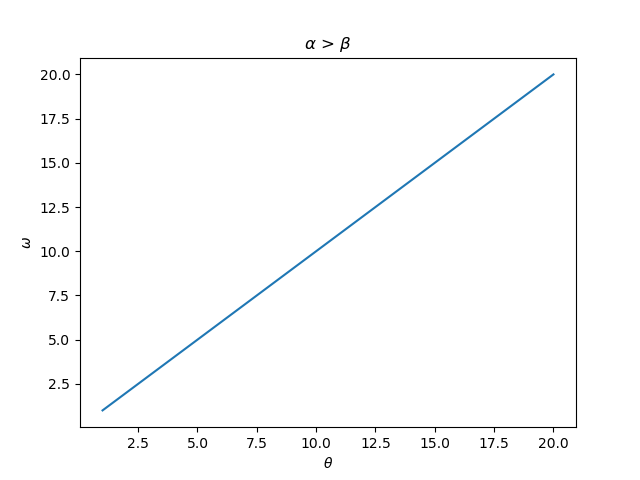由此可见，打印特殊符号需要知道特殊符号的英文名称，在前面加上转义符反斜杠，再用一对美元符号包起来即可。
接下来，我们尝试打印下标和上标。下标需要加"_"，上标需要加"^"，这跟latex语法完全一样。
import matplotlib.pyplot as plt

x = [i+1 for i in range(20)]
y = x
plt.figure()
plt.title(r'$\alpha_i$ > $\beta_i$')  # 打印α_i > β_i
plt.xlabel(r'$\theta^2$')  # 打印θ^2
plt.ylabel(r'$\omega^n$')  # 打印ω^n
plt.plot(x, y)
plt.show()

我们看看效果：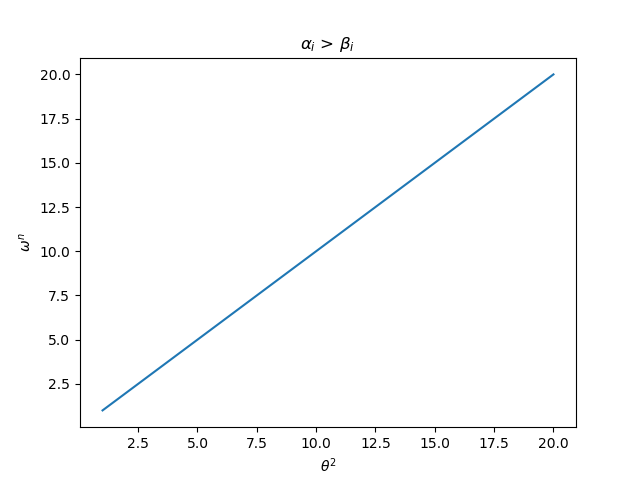更多符号对应字母请见下图：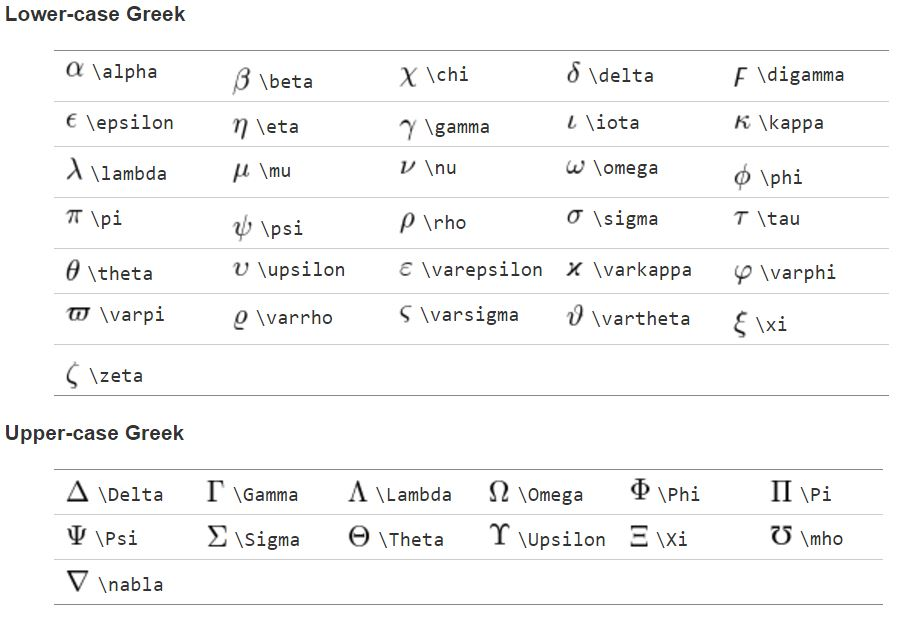2、制作图例，legend函数
import matplotlib.pyplot as plt
from math import sin, cos, exp

x = [(i+1)/100 for i in range(1000)]
y1 = [sin(i) for i in x]
y2 = [cos(i) for i in x]
y3 = [exp(-i) for i in x]

plt.figure()
plt.plot(x, y1)
plt.plot(x, y2)
plt.plot(x, y3)

# 分别对应y1，y2，y3标志图例，注意e^(-x)要加大括号表示(-x)是一个整体，都是上标
plt.legend(['sin(x)', 'cos(x)', '$e^{-x}$'])
plt.show()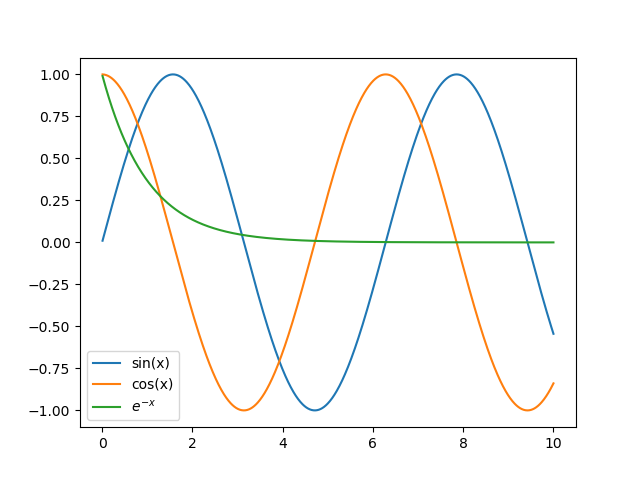在文章最后附上参考链接~ https://matplotlib.org/users/mathtext.html
展开全文上标下标
• 利用python的plt绘制多种柱状图，进行数据可视化
首先导入必要的包
import pandas as pd
import numpy as np
import matplotlib.pyplot as plt

# 在jupyter画图时，添加下面三行，设置中文字体，防止中文乱码
%matplotlib inline
plt.rcParams['font.sans-serif']=['SimHei'] #用来正常显示中文标签
plt.rcParams['axes.unicode_minus']=False #用来正常显示负号

绘制并列柱状图(以两个柱子为例) 1.1 利用pandas绘制
data = np.random.rand(10,2)  # 生成数据
idx = [i for i in range(3, 13)]  # 可以设置索引
df = pd.DataFrame(data, columns=['列1', '列2'], index=idx)
# df = pd.DataFrame(data, columns=['列1', '列2'])   # 使用默认索引
ax = df.plot(kind='bar')
ax.set_xlabel('年份')  # 设置x轴标签
ax.set_ylabel('分数') # 设置y轴标签
fig = ax.get_figure()  # 用于保存图片
fig.savefig('pic.png')  # 保存为png格式1.2 利用plt直接绘制
name_list = ['apple', 'banana', 'car', 'dog'] # 设置横坐标的刻度名称
y_list1 = [0.63,  0.96, 0.73,  0.9]     # 纵坐标
y_list2  = [0.51,  0.87, 0.83,  0.81]     # 纵坐标
x =np.arange(len(name_list))
width = 0.25  # 设置柱子的宽度和间隔

# bar参数说明:前两个分别为x和y; width: 表示柱子宽度; label: 图例名称,所代表的类别; fc: 设置颜色
plt.bar(x, y_list1, width=width, label='列1',fc = 'deepskyblue')
x = x + width
#plt.bar(x, y_list2, width=width, label='列2', fc = 'orange', tick_label = name_list)
# 下两句的效果和上面这一句差不多,只是上面一句设置的x轴标签不是居中的
plt.bar(x, y_list2, width=width, label='列2', fc = 'orange')
plt.xticks(x-width/2, tick_label)#显示x坐标轴的标签,并调整位置，使其落在两个直方图中间位置
plt.legend()              # 显示图例
plt.title("利用plt绘制")  # 设置表格标题
plt.savefig("pic2.png")  # 保存图片
plt.show()                # 展示图片
# 需要注意的是, 保存图片的时候, plt.savefig()应该放在plt.show()前面,否则保存的图片将会是空白图片.
# 个人猜测原因可能是调用show之后, 就会把这个对象清空吧.2. 堆叠图 2.1 利用pandas画堆叠图
data = np.random.rand(10,3)  # 生成数据
idx = [i for i in range(3, 13)]  # 可以设置索引
df = pd.DataFrame(data, columns=['列1', '列2', '列3'], index=idx)
# df = pd.DataFrame(data, columns=['列1', '列2'])   # 使用默认索引
ax = df.plot(kind='bar', stacked=True)
ax.set_xlabel('年份')  # 设置x轴标签
ax.set_ylabel('分数') # 设置y轴标签
fig = ax.get_figure()  # 用于保存图片
#fig.savefig('pic.png')  # 保存为png格式

显然，和上面用pandas画并列柱状图的方法是一样的，只是在plot的时候，把参数设置为 stacked=True参考链接与推荐链接
plt画图pandas中文网可视化的教学与例子pandas中文网中关于plot的参数说明matplotlib.pyplot的官方介绍与应用举例，参数说明菜鸟教程里的Matplotlib 教程（中文的）
事实上，对于一些很出名的库，直接去官网上查找教程，反而是最简单且最详细的，甚至还有一些有中文教程，提供给我们阅读。 以上，如有错误，还望指出。
展开全文python
• 使用plt显示nii_1和nii_2中的slice for i in range(len(low_data)): fig = plt.figure() fig.add_subplot(1,2,1) plt.imshow(low_data[i],cmap='gray') fig.add_subplot(1,2,2) plt.imshow(high_data[i],...
Extracting data:
1.nibable
import nibabel as nib
nii_1 = nii_1.dataobj #obtain the nii data
nii_2 = nii_2.dataobj #obtain the nii data
import SimpleITK as sitk
image_array = sitk.GetArrayFromImage(image) 
使用opencv显示nii_1和nii_2中的slice
def nothing(emp):
pass

def visualize(name,frames):
cv2.namedWindow(name,0)
#cv2.resizeWindow(name, 800, 600)
loop_flag = 0
pos = 0
cv2.createTrackbar('time', name, 0, frames, nothing)
while True:
if cv2.waitKey(1) & 0xFF == ord('q'):
cv2.destroyAllWindows()
break
pos = cv2.getTrackbarPos('time', name)
img1_new = nii_1[pos]
img2_new = nii_2[pos]
img_new = np.hstack([img1_new,img2_new])
cv2.imshow(name, img_new)

visualize('img', len(high_data)-1)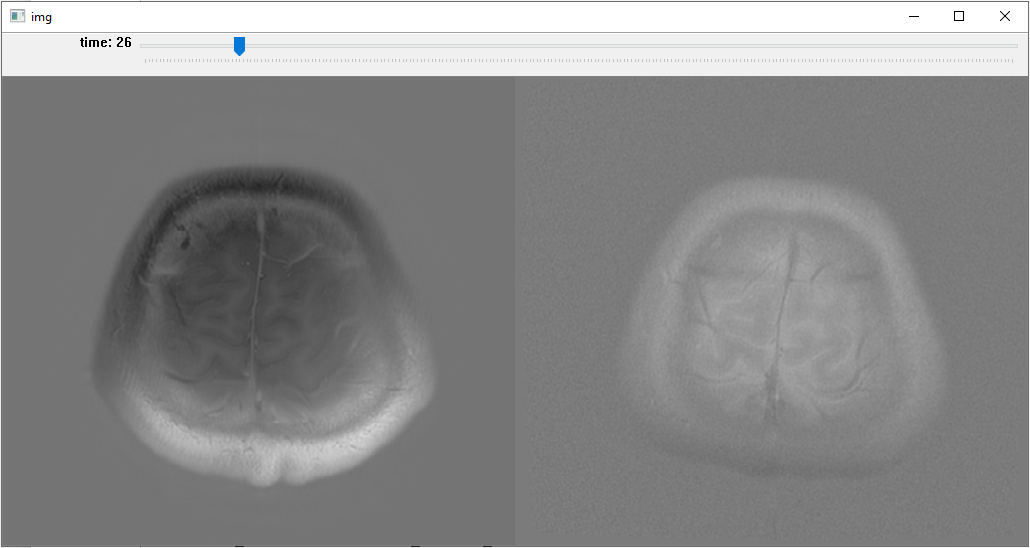使用plt显示nii_1和nii_2中的slice
 for i in range(len(low_data)):
fig = plt.figure()
plt.imshow(low_data[i],cmap='gray')
plt.imshow(high_data[i],cmap='gray')
plt.pause(1)
plt.close()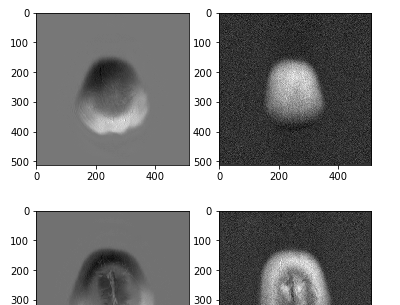展开全文opencv matplotlib
• savefig要写在show前面 不然保存的就是空白图片 文件名是新命名 路径斜杠直接复制记得取反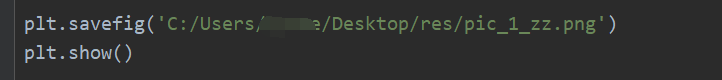savefig要写在show前面  不然保存的就是空白图片
文件名是新命名
路径斜杠直接复制记得取反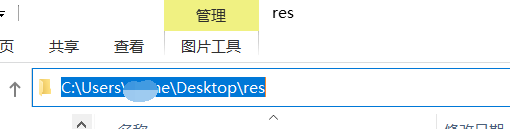展开全文• 使用可视化库matplotlib绘图时，plt.show()过后只出现<Figure size 640x480 with 1 Axes>而没有生成图片 解决： 可以在前面添加 plt.figure()创建画布。 或者 导入库之后添加以下代码即可 %...
• 一、可视化库导入 %matplotlib inline import matplotlib.pyplot as plt 二、显示彩色图像 plt.figure(figsize=(15,10)) plt.imshow(img) plt.show() 前提是，img是以彩色图像读入的 如果采用opencv读入的...
• import matplotlib.pyplot as plt %matplotlib inline image = cv2.imread('1000/wirebroken_0001_1.jpg') image = cv2.cvtColor(image, cv2.COLOR_BGR2RGB) def visualize(image): plt.figure(figsize=(10,10)) ...python opencv 计算机视觉
• 数据可视化函数plt.plot(x,y,ls,lw,c,marker,markersize,markeredgecolor,markerfacecolor,label) 设置画线的标准和形式 参数说明： **x：**横坐标；**y：**纵坐标；**ls或linestyle：**线的形式（‘-’，‘–’，...
• 前一篇文章讲述了数据预处理、Jieba分词和文本聚类知识，这篇文章主要介绍Matplotlib和Pandas扩展包绘图的基础用法，同时引入Echarts技术，该技术主要应用于网站可视化展示中。本文内容以实例为主，给读者最直观的...Python Matplotlib Pandas Echarts
• 条形图绘制plt.bar() 上一篇我们讲到了折线图的绘制，下来来说一下条形图绘制… 码上… import matplotlib.pyplot as plt import numpy as np N = 5 y = [20, 30, 10, 25, 15] index = np.arange(N) plt.bar...python 机器学习 人工智能
• 一、fig, ax = plt.subplots()的作用？ 二、参数的含义？ 三、怎么在一个图上排列多个子图？ 四、怎么把多个子图一起合并到一个图上？ 《Python for Data Analysis》 2nd Edition 一、fig, ax = plt.subplots()...python fig 格式
• 生成的图片保存后发现左边少了一部分 需要将代码中添加参数 bbox_inches = 'tight' plt.savefig('XGBoost_feature_importance.png', bbox_inches = 'tight') plt.show()python
• 数据可视化 基本概念 数据可视化是指借助于图形化的手段，清晰、快捷有效的传达与沟通信息。同时，也可以辅助用户做出相应的判断，更好的去洞悉数据背后的价值。 字不如表，表不如图。 观察号码的频率，每个号码...Matplotlib
• 使用如下代码保存使用plt.savefig保存生成的图片时，结果打开生成的图片却是一片空白。 例如： # 导入可视化工具包 import matplotlib.pyplot as plt %matplotlib inline # 颜色和标签列表 colors_list = ['...python
• plt.plot(x, y, ls="-.",color=“r”,marker =",", lw=2, label=“plot figure”) x：x轴数值 y：y轴数值 ls：折线图的线条风格 color：颜色 marker：标记样式 lw：折线图的线条宽度 label：标记图形内容的标签文本...matplotlib plot
• t-SNE可视化图像特征easy sample(使用sklearn包):Scatter图Grid图Example t-SNE（t-Distributed Stochastic Neighbor Embedding）是一种非线性降维技术，主要用途为对高维数据进行可视化。 easy sample(使用sklearn...python 计算机视觉
• 基本都是plt.savefig和plt.show()的顺序问题，但是我的顺序并没有错， 同样也输出为空白，我是从这里找到的解决方法： 将matplotlib子图图形保存到图像文件 同时英文好的大佬也可以看看这个，虽然我看不懂，但是这...
• 通常呢，大家绘制图片，立马想到的是matplotlib这个包 ，也就是说，你只需调用 .plot() 方法，即可快速绘制简单的 pd.DataFrame 或 pd.Series。如下： import matplotlib.pyplot as plt import pandas as pd import ...
• 可视化模拟正弦余弦在子图显示 import matplotlib.pyplot as plt import numpy as np plt.rcParams['font.sans-serif'] = ['simhei'] plt.rcParams['axes.unicode_minus'] = False #生成模拟数据 x = np.arange...python
• import matplotlib.pyplot as plt import numpy as np # 防止乱码 mpl.rcParams["font.sans-serif"] = ["SimHei"] mpl.rcParams["axes.unicode_minus"] = False # 生成数据 x = [1,2,3,4,5] y1 = [6,10,4,5,1] y2 ...python matplotlib bar 堆积图
• 跟着小象学院学python，今天学习了读取csv数据输出可视化图片 老师的例子是window下的，我在linux下，系统字体跟例子的肯定不一样 原先其他输出可视化是用单独部件设置字体，这里输出用了plot()整体输出，没有...python pandas matplotlib
• Python可视化-WordCloud生成云词图片 首先建一个记事本，写入你想要生成云词图片的文章。 生成的云图 ##完整示例代码 import jieba.analyse ##jieba库 用于分割中文词语 from PIL import Image,ImageSequence ...Python wordcloud 云词 matplotlib
• python实现数据可视化I Feel: 我觉得： In today’s digital world data has become as important as air. Machines & humans both are literally breathing in & breathing out data, data & data…. ...python 机器学习 数据分析
• TensotFlow 应用实例：06-使用matplotlib可视化训练过程 本文是我在学习TensotFlow 的时候所记录的笔记，共享出来希望能够帮助一些需要的人。 import tensorflow as tf import numpy as np import matplotlib....实例 应用
• 数据可视化可以让我们很直观的发现数据中隐藏的规律，察觉到变量之间的互动关系，可以帮助我们更好的给他人解释现象，做到一图胜千文的说明效果。 常见的数据可视化库有: matplotlib 是最常见的2维库，可以算作...python 数据分析
• colormap = [[] for _ in range(len(data))] for i in range(len(data)): colormap[i] = lab2seg[cls[j]][data[i, -1]] #设置图片大小 plt.figure(figsize=(10,10)) ax = plt.subplot(111, projection='3d') #设置...
• 来源：大邓和他的Python数据可视化可以让我们很直观的发现数据中隐藏的规律，察觉到变量之间的互动关系，可以帮助我们更好的给他人解释现象，做到一图胜千文的说明效果。常见的数据可视化库有:...编程语言 数据挖掘 数据分析
• 之前写了用pyecharts画词云，今天我们来看一下用python的wordcloud库画词云。 wordcloud接收的数据有两种格式，一种是纯文本的...import matplotlib.pyplot as plt ### 纯文本数据 data = ['重庆','上海','北京','...wordcloud 例子...